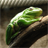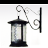# Slope + Speed + Distance + Impedance = Travel Time

3587
6
08-11-2011 10:19 PMby
New Contributor
Hi.

I am trying to calculate a shortest path based on travel time through my pedestrian and bicycle network.

However, I want to incorporate:  slope, travel speed, distance, pedestrian impedance (sidewalks), and bicycle impedance (safest route) into an equation to help calculate travel time through the network.

I'm thinking that the easiest thing to do is create an equation in the  Field Calculator that incorporates these variables and populate a new field (Travel time).

I was thinking about modifying Tobler's equation http://mapaspects.org/courses/gis-and-anthropology/workshop-2009-viewshed-and-cost-distance/ii-cost-...

Just not sure exactly how to formulate the equation? Any thoughts?
Tags (2)
6 Repliesby
Occasional Contributor II
I do not think this is a question for network analyst. This is for spatial analyst on raster analysis. The results will therefore depend on the resolution of the raster. after raster analysis, you can bring it back to network analyst.byMVP Esteemed Contributor
Seems like there have been discussions about incorporating slope into an impedance value in past; you may want to do a search of the forum, including the old one.

Perhaps Jay S or another member of Team Network Analyst will chime in for this one.
can't wait to retire....Regular Contributor III
Agreed. The slope might be a valuable parameter to use as a cost. The current workflow I would suggest to implement is to produce the slope surface and then transfer those values to the vector street features as was suggested above.by
New Contributor
Hi all.

I have a vector street network and within the network, I have calculated the following for each line segment: slope, an impedance value, distance, and a travel speed (average).

My hope was to devise some type of formula in the field calculator that incorporates these variables and populate a new field which, I could then use as my cost field (travel time) when creating a new network dataset.

I haven't ran across this is the forums (new or old), but I will double check.

Thanks!by
Occasional Contributor II
It is very tempting to use such formulas. But if your speed values are field measured values, then it is already a function of slope, distance and impedance (whatever that might be), so you would just be overdoing it.

if your speed is a speed limit regulation value, then you can make a linear combination to keep it simple. For travel time,

1. upslope will have a reduce the speed and hence more travel time (so positive coefficient)
2. high speed will reduce travel time (so negative coefficient)
3. long distance will increase travel time (so positive coefficient)
4. more impedance will increase travel time (so positive coefficient)

Now you can make linear combinations as it might suit your requirements, you can add weights to them etc.byMVP Esteemed Contributor
Here's one.
can't wait to retire....